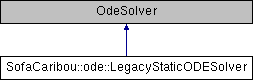Caribou
SofaCaribou::ode::LegacyStaticODESolver Class Reference
Inheritance diagram for SofaCaribou::ode::LegacyStaticODESolver:## Public Member Functions

SOFA_CLASS (LegacyStaticODESolver, sofa::core::behavior::OdeSolver)

void solve (const sofa::core::ExecParams *params, double dt, sofa::core::MultiVecCoordId xResult, sofa::core::MultiVecDerivId vResult) override

auto iteration_times () const -> const std::vector< UNSIGNED_INTEGER_TYPE > &
List of times (in nanoseconds) that each Newton-Raphson iteration took to compute in the last call to Solve().

auto squared_residuals () const -> const std::vector< FLOATING_POINT_TYPE > &

auto squared_initial_residual () const -> const FLOATING_POINT_TYPE &

auto iterative_linear_solver_squared_residuals () const -> const std::vector< std::vector< FLOATING_POINT_TYPE >> &

auto iterative_linear_solver_squared_rhs_norms () const -> const std::vector< FLOATING_POINT_TYPE > &

double getVelocityIntegrationFactor () const override
Given a displacement as computed by the linear system inversion, how much will it affect the velocity. More...

double getPositionIntegrationFactor () const override
Given a displacement as computed by the linear system inversion, how much will it affect the position. More...

virtual double getPositionIntegrationFactor (double dt) const

double getIntegrationFactor (int inputDerivative, int outputDerivative) const override
Given an input derivative order (0 for position, 1 for velocity, 2 for acceleration), how much will it affect the output derivative of the given order. More...

double getIntegrationFactor (int inputDerivative, int outputDerivative, double dt) const

double getSolutionIntegrationFactor (int outputDerivative) const override
Given a solution of the linear system, how much will it affect the output derivative of the given order.

double getSolutionIntegrationFactor (int outputDerivative, double dt) const

## Protected Attributes

Data< unsigned > d_newton_iterations
INPUTS.

Data< double > d_correction_tolerance_threshold

Data< double > d_residual_tolerance_threshold

Data< bool > d_shoud_diverge_when_residual_is_growing

Data< bool > d_warm_start

Data< bool > d_converged
OUTPUTS Whether or not the last call to solve converged.

## ◆ getIntegrationFactor()

 double SofaCaribou::ode::LegacyStaticODESolver::getIntegrationFactor ( int inputDerivative, int outputDerivative ) const
inlineoverride

Given an input derivative order (0 for position, 1 for velocity, 2 for acceleration), how much will it affect the output derivative of the given order.

This method is used to compute the compliance for contact corrections. For example, a backward-Euler dynamic implicit integrator would use: Input: x_t v_t a_{t+dt} x_{t+dt} 1 dt dt^2 v_{t+dt} 0 1 dt

If the linear system is expressed on s = a_{t+dt} dt, then the final factors are: Input: x_t v_t a_t s x_{t+dt} 1 dt 0 dt v_{t+dt} 0 1 0 1 a_{t+dt} 0 0 0 1/dt The last column is returned by the getSolutionIntegrationFactor method.

## ◆ getPositionIntegrationFactor()

 double SofaCaribou::ode::LegacyStaticODESolver::getPositionIntegrationFactor ( ) const
inlineoverride

Given a displacement as computed by the linear system inversion, how much will it affect the position.

This method is used to compute the compliance for contact corrections For Euler methods, it is typically dt².

## ◆ getVelocityIntegrationFactor()

 double SofaCaribou::ode::LegacyStaticODESolver::getVelocityIntegrationFactor ( ) const
inlineoverride

Given a displacement as computed by the linear system inversion, how much will it affect the velocity.

This method is used to compute the compliance for contact corrections For Euler methods, it is typically dt.

## ◆ iterative_linear_solver_squared_residuals()

 auto SofaCaribou::ode::LegacyStaticODESolver::iterative_linear_solver_squared_residuals ( ) const -> const std::vector> &
inline

The list of squared residual norms (||r||^2) of every iterative linear solver iterations, for each newton iterations of the last solve call.

## ◆ iterative_linear_solver_squared_rhs_norms()

 auto SofaCaribou::ode::LegacyStaticODESolver::iterative_linear_solver_squared_rhs_norms ( ) const -> const std::vector &
inline

List of squared right-hand side norms (||b||^2) of every newton iterations before the call to the solve method of the iterative linear solver.

## ◆ squared_initial_residual()

 auto SofaCaribou::ode::LegacyStaticODESolver::squared_initial_residual ( ) const -> const FLOATING_POINT_TYPE &
inline

The initial squared residual (||r0||^2) of the last solve call.

## ◆ squared_residuals()

 auto SofaCaribou::ode::LegacyStaticODESolver::squared_residuals ( ) const -> const std::vector &
inline

The list of squared residual norms (||r||^2) of every newton iterations of the last solve call.

The documentation for this class was generated from the following files:
• /home/jnbrunet/sources/caribou/src/Plugin/Ode/LegacyStaticODESolver.h
• /home/jnbrunet/sources/caribou/src/Plugin/Ode/LegacyStaticODESolver.cpp